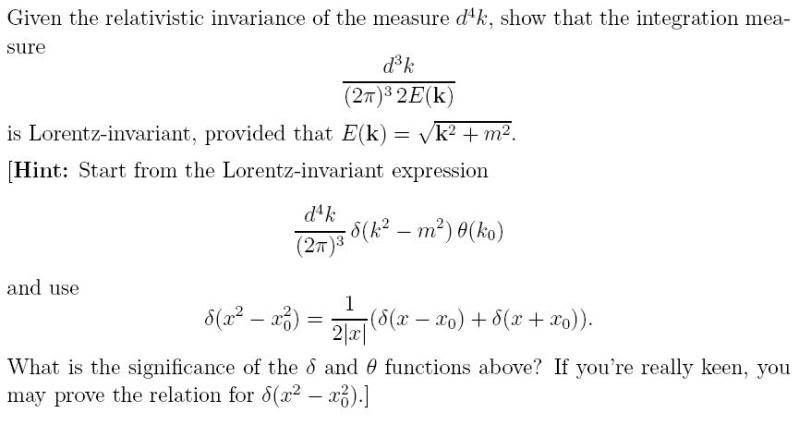# Proving the Lorentz invariance of an integration measure? QFT related?So, first off, I'm thinking Lorentz invariant quantities are the same in any inertial frames S and S' regardless of their relative velocity.

I'm thinking I need to show that
$\frac{d^3k}{(2\pi)^32E(\vec{k})} = \frac{d^3k'}{(2\pi)^32E'(\vec{k'})}$ where the primed & unprimed quantities denote different frames.

We also have $E(\vec{k}) = \sqrt{\vec{k}^2 + m^2}$ in the denominator. This isn't Lorentz invariant - the mass term is, but the 3 - momentum $\vec{p} = \hbar \vec{k}$ is not, therefore E is not.

$E \neq E'$ where $E'(\vec{k'}) = \sqrt{\vec{k}'^2 + m^2}$.

This is making me think that neither E nor d3k are Lorentz invariant, so that when they are used in this fraction, the Lorentz invariance from each one somehow cancels out overall.

Then we come to the hint. I 'm really not sure what to make of this, I mean, I can write $\delta(x^2 - x_0^2) = \frac{1}{2|x|}(\delta(x-x_0) + \delta(x+x_0))$ with k's and m's: $\delta(k^2 - m^2) = \frac{1}{2|k|}(\delta(k-m) + \delta(k+m))$
to get an apparently Lorentz invariant expression: $\frac{d^4k}{(2\pi)^3}\delta(k^2 - m^2)\theta(k_0) = \frac{d^4k}{(2\pi)^3}\frac{1}{2|k|}(\delta(k-m) + \delta(k+m))\theta(k_0)$
but I'm not sure where this gets me, other than guessing that
$\delta(k^2 - m^2)\theta(k_0)$ is also Lorentz invariant seeing as I am told d4k is.

I have no idea how to proceed with this. I don't know what to make of the delta functions either.

vela
Staff Emeritus
Homework Helper
You should recognize that $d^4k\ \delta(k^2-m^2)\theta(k_0)$ is manifestly Lorentz invariant because $k^2 = k^\mu k_\mu$ is invariant.

The idea here is to note that $k^2-m^2 = k_0^2-\mathbf{k}^2-m^2$ and to do the integral over k0.

Intuitively, you can think of the volume $d^3k$ being reduced by a factor $\gamma$ due to length contraction while E(k) is reduced by the same factor due to time dilation, so their ratio is invariant.

You should recognize that $d^4k\ \delta(k^2-m^2)\theta(k_0)$ is manifestly Lorentz invariant because $k^2 = k^\mu k_\mu$ is invariant.

right, so, if I'm not mistaken, $k^\mu = (k_0, k_1, k_2, k_3)$ and $k_\mu = (k_0, -k_1, -k_2, -k_3)$. So, $k^2 = k^\mu k_\mu = (k_0, k_1, k_2, k_3).(k_0, -k_1, -k_2, -k_3) = k_0^2 - k_1^2 -k_2^2 -k_3^2 = k_0^2 - \vec{k}^2$.
Is there some reason why I should instantly recognise this as an invariant quantity?
Do you always get an invariant quantity if you take any 4-vector and do this with it?
Also, what am I supposed to make of the $\theta(k_0)$ or the d4k? What about them says Lorentz invariant?

The idea here is to note that $k^2-m^2 = k_0^2-\mathbf{k}^2-m^2$ and to do the integral over k0.

I'm meant to be computing $\frac{1}{(2\pi)^3}∫ \delta(k_0^2 - \vec{k}^2 - m^2)\theta(k_0)d^4k$ right?
I'm struggling to be change the $d^4k$ into something involving a dk0.
Can I start off by saying $dk^\mu = (dk_0, dk_1, dk_2, dk_3)$?

Actually, can I say that $d^4k = d^3k dk_0$?

Last edited:
vela
Staff Emeritus
Homework Helper
right, so, if I'm not mistaken, $k^\mu = (k_0, k_1, k_2, k_3)$ and $k_\mu = (k_0, -k_1, -k_2, -k_3)$. So, $k^2 = k^\mu k_\mu = (k_0, k_1, k_2, k_3).(k_0, -k_1, -k_2, -k_3) = k_0^2 - k_1^2 -k_2^2 -k_3^2 = k_0^2 - \vec{k}^2$.
Is there some reason why I should instantly recognise this as an invariant quantity?
Do you always get an invariant quantity if you take any 4-vector and do this with it?
Yes, the product of any two four-vectors, including a four-vector with itself, is an invariant. It's analogous to the regular dot product being invariant under rotations.
Also, what am I supposed to make of the $\theta(k_0)$ or the d4k? What about them says Lorentz invariant?
Think about the coordinate transformation $k'^\mu = \Lambda^\mu{}_\nu k^\nu$ where $\Lambda^\mu{}_\nu$ is a Lorentz transformation. How are the volume elements related? What about the signs of k0 of k'0?
I'm meant to be computing $\frac{1}{(2\pi)^3}∫ \delta(k_0^2 - \vec{k}^2 - m^2)\theta(k_0)d^4k$ right?
I'm struggling to be change the $d^4k$ into something involving a dk0.
Can I start off by saying $dk^\mu = (dk_0, dk_1, dk_2, dk_3)$?

Actually, can I say that $d^4k = d^3k dk_0$?
Yes, $d^4k = d^3k\:dk_0$